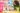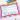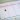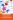# How To Make The Most Of Printable Math Games

Printable math games should have a place in your class as they provide practice that doesn't feel like a chore. Here are 3 games to help make math fun.Who needs printable math games? I’m a fan of using games in the classroom for all subjects, but I see a lot of math haters. Math card games and other math games can help turn that around. It provides extra practice that doesn’t feel like a chore. Some games can even be used as informal assessments, which can help get a sense of where kids are if they tend to stress out or freeze up on math tests.

While you may think of games that practice the basic functions, like addition, subtraction, multiplication, and division, classroom math activities and games can cover lots of areas of math, including

• Shapes
• Estimating
• Place value
• Counting on
• Time
• Length
• Area

And so many more.## Printable math games for school or home

Printable math games should have a place in your class whether as whole class activities, small group activities, or even individual activities. You can assign classroom math activities for everyone to do at a certain time, have them available in your math center or have them as an option for academic choice time. Many printable math games are also easy to send as homework for additional practice.

Here are three games and two resources to help make math fun.

### Knock out

Each player needs a printed number strip 1–10 and a pencil or highlighter. (If you laminate your number strips, students can use the number strip again and again with a dry erase marker.) You’ll need two dice for the game.

The first player rolls the dice and can use any operation on their two numbers. For example, if the student rolled a 2 and a 4, they have these choices:

2 + 4 = 6
4 – 2 = 2
2 ⨉ 4 = 8
4 ÷ 2 = 2

The student can then decide which number to “knock out.” They cross out or color in the number that they decide on. Sometimes, there are many choices, sometimes few. If the only options are numbers students have already knocked out, they have to pass that turn. The first person to knock out all their numbers wins.

After the game, ask students to think about which numbers are easiest to knock out and which are the hardest. Does that affect the decisions they make the next time they play?

Create a set of dominoes. On one side of each domino, have a number. On the other side, have an addition problem. Students have to add the numbers to get the sum to match it to another domino. You could set up the same thing for subtraction, multiplication, or division.

### Place values

You will need one die per pair playing. Each student will need a recording sheet with thousands, hundreds, tens, and ones columns labeled. (Alternately, have students set up a chart with these place values in a notebook.) The goal is to create the largest number (you can mix it up by having students create the smallest number.)

• The first student rolls the die and decides where to put it.
• Then the next student does the same.
• Each student rolls 4 times to fill in the chart.
• The student with the larger number (or smaller if that is the parameter you set) wins.

Ask students to identify the largest and smallest possible number they could have created using the numbers they rolled.## Printable math games

Looking for some games you can print and use? Get 3 FREE Math Games to use in your classroom or send home for kids to use for practice. Fill in the form below to grab them now!

### FREE Math Games & ActivitiesIf you want more than that, Fun Math Worksheets and Games has you covered for a wide variety of topics including:

• Number
• Shape
• Mental math
• Measurement
• Space
• Chance and data

You get dice games, paper and pencil games, card games, and calculator games plus puzzles, task cards, dominoes, and more. Check out everything covered and get your copy here:### FREE Math Games & Activities## Why I Love Task Cards For Teaching Multiplication

Teaching multiplication goes beyond having children memorize multiplication tables. To build math fluency, try these best ways to teach multiplication.

## 6 Great Ideas For Teaching Place Value

Teaching place value is important for students. Place value helps us to read whole numbers into the millions and beyond. Try these place value activities.